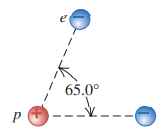### Solution Found!# Solved: If two electrons are each 1.50 X 10-10 m from a

Chapter 21, Problem 37E

#### (choose chapter or problem)

Get Unlimited Answers! Check out our subscriptions

QUESTION:

Problem 37E

If two electrons are each $$1.50 \times 10^{-10}$$ m from a proton, as shown in Fig. E21.37, find the magnitude and direction of the net electric force they will exert on the proton.Equation Transcription:Text Transcription:

1.50x10^-10

65.0degC

Step 1 of 2

Electrons are negatively charged, whereas the proton is positively charged. Let us have a look at the following figure to understand the direction of net electric force the electrons would exert on the proton.Become a subscriber and get unlimited answers!